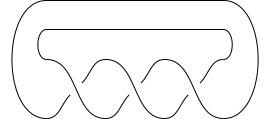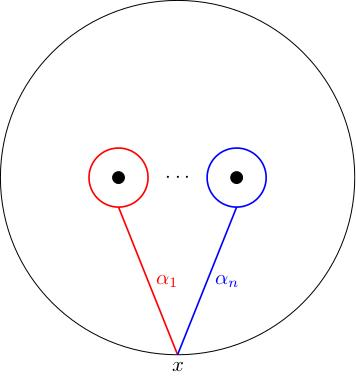# 6.03 Braids: the Wirtinger presentation

Below the video you will find notes and some pre-class questions. Once again, sorry for the gurgling background noises: I can't turn off my office radiator.

# Notes

(0.00) Let $$B$$ be an $$n$$-strand braid inside $$D^2\times[0,1]$$. If we take the quotient space $$D^2\times S^1=(D^2\times[0,1])/\sim$$, $$(x,0)\sim(x,1)$$, then the braid closes up to become a collection of embedded circles $$C_B$$ in $$D^2\times S^1$$ (because the component paths $$B_k(t)$$ start and end in the set of points $$z_1,\ldots,z_n$$). This is called the braid closure $$C_B$$ of $$B$$.

Here is an example: the braid closure of the 2-strand braid $$\sigma_1^3$$ is the trefoil knot:(1.59) Let $$X_B=(D^2\times S^1)\setminus C_B$$ denote the complement of $$C_B\subset D^2\times S^1$$. Let $$x=[1,0]\in(D^2\times[0,1])/\sim$$ (we are thinking of $$D^2\subset\mathbf{C}$$, so $$1\in D^2$$ makes sense). We have \begin{equation} \label{eq:knotcomp1} \pi_1(X_B,x)=\langle\alpha_1,\ldots,\alpha_n,g\ |\ g\alpha_kg^{-1} =B(\alpha_k)\mbox{ for }k=1,\ldots,n\rangle. \end{equation} Here, $$g$$ is the loop $$x\times S^1$$ and, for $$k\in\{1,\ldots,n\}$$, $$\alpha_k$$ is the element of $$\pi_1(D^2\setminus\{z_1,\ldots,z_n\}$$ given by the loop in the figure below and $$B(\alpha_k)$$ denotes the Artin action of $$B$$ on $$\alpha_k\in\pi_1(D^2\setminus\{z_1,\ldots,z_n\}\cong\mathbf{Z}^{\star n}$$.
(3.01) The space $$X_B$$ is the mapping torus of the homeomorphism $Art_B\colon D^2\setminus\{z_1,\ldots,z_n\}\to D^2\setminus\{z_1,\ldots,z_n\},$ so the lemma follows from the result we proved earlier which gave a presentation for the fundamental group of a mapping torus.Consider the 2-strand braid $$\sigma_1$$ (whose braid closure is an unknot). We have $\sigma_1(\alpha)=\alpha\beta\alpha^{-1},\quad\sigma_1(\beta)=\alpha,$ so the presentation for $$\pi_1(X_{\sigma_1})$$ is: $\langle\alpha,\beta,g|g\alpha g^{-1}=\alpha\beta\alpha^{-1},g\beta g^{-1}=\alpha\rangle.$

(8.44) If we embed $$D^2\times S^1$$ as the standard solid torus in $$\mathbf{R}^3$$ then the complement of the braid closure $$C_B\subset \mathbf{R}^3$$ has \begin{equation} \label{eq:knotcomp2} \pi_1(\mathbf{R}^3\setminus C_B)=\langle\alpha_1,\ldots,\alpha_k\ |\ \alpha_k=B(\alpha_k),\ k=1,\ldots,n\rangle \end{equation} where $$B(\alpha_k)$$ is the Artin action of $$B$$ on the free group $$\langle\alpha_1,\ldots,\alpha_k\rangle$$.
(A homotopy retract of) the complement $$\mathbf{R}^3\setminus C_B$$ is obtained from $$X_B$$ by attaching a 2-cell along the circle $$x\times S^1$$, which adds the relation $$x=1$$ to the presentation from the lemma, yielding the desired presentation.

(11.26) Consider the 2-strand braid $$\sigma_1$$ (whose braid closure is an unknot). We have $\pi_1(X_{\sigma_1})=\langle\alpha,\beta,g|g\alpha g^{-1}=\alpha\beta\alpha^{-1},g\beta g^{-1}=\alpha\rangle$ so the Wirtinger presentation is obtained by setting $$g=1$$: $\langle\alpha,\beta|\alpha=\alpha\beta\alpha^{-1},\beta=\alpha\rangle.$ We can simplify this to just get $$\langle\alpha\rangle$$, so the fundamental group is $$\mathbf{Z}$$.

I said that this allows us to compute the fundamental group of any knot complement: this is because one can show that any knot is isotopic to a braid closure; a proof of this was first written down by Alexander (1923, A lemma on a system of knotted curves'') and it is quite readable.

# Pre-class questions

1. The video claimed that "any braid gives a knot by taking the braid closure". Why was this claim false? What should I have said instead?